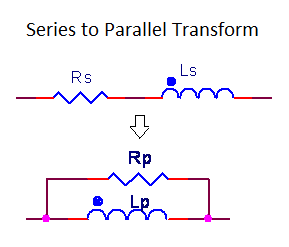# RL Series to Parallel Impedance Transform Calculator

This CalcTown calculator calculates the equivalent resistance and inductance values in parallel for a given series combination of the same.

Serial/parallel R-L networks are ubiquitous in low frequency and RF designs.  The necessity to view these simple circuits in both serial and parallel forms often recurs, especially during analysis.

#### Result

Ω
HWhere,

Rs = Resistance in series

Rp = Resistance in parallel

f = Operating Frequency

Q = Quality factor

Ls = Inductance in series

Lp = Inductance in parallel